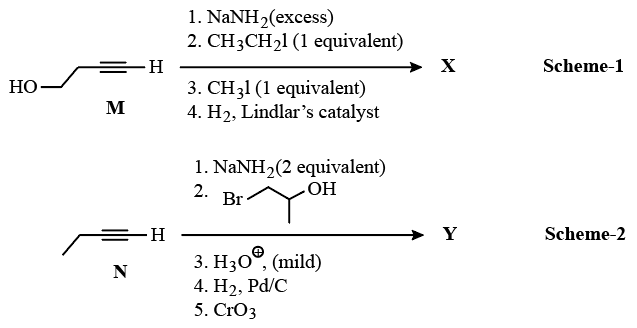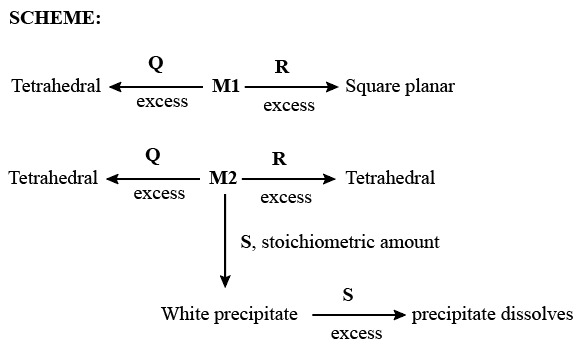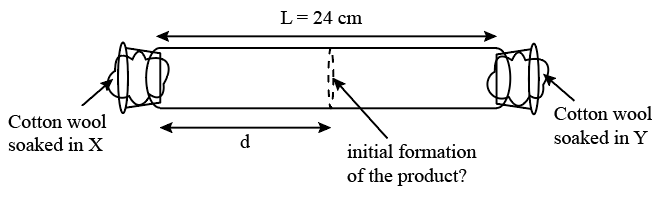Instructions

Schemes 1 and 2 describe sequential transformation of alkynes M and N. Consider only the major products formed in each step for both the schemes.Question 31

Question 32

# The correct statement with respect to product Y is

Instructions

An aqueous solution of metal ion M1 reacts separately with reagents Q and R in excess to give tetrahedral and square planar complexes, respectively. An aqueous solution of another metal ion M2 always forms tetrahedral complexes with these reagents. Aqueous solution of M2 on reaction with reagent S gives white precipitate which dissolves in excess of S. The reactions are summarized in the scheme given below:Question 33

Question 34

# Reagent S is

Instructions

X and Y are two volatile liquids with molar weights of $$10 g mol^{-1}$$ and $$40 g mol^{-1}$$ respectively. Two cotton plugs, one soaked in X and the other soaked in Y, are simultaneously placed at the ends of a tube of length L = 24 cm, as shown in the figure. The tube is filled with an inert gas at 1 atmosphere pressure and a temperature of 300 K. Vapours of X and Y react to form a product which is first observed at a distance d cm from the plug soaked in X. Take X and Y to have equal molecular diameters and assume ideal behaviour for the inert gas and the two vapours.Question 35

Question 36

# The experimental value of d is found to be smaller than the estimate obtained using Graham’s law. This is due to

Instructions

For the following questions answer them individually

Question 37

Question 38

Question 39

Question 40

OR